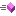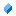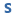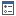﻿ NGraphicsPath Class Members
Nevron .NET Vision
Nevron.GraphicsCore Namespace / NGraphicsPath Class
Fields Properties Methods

In This Topic
NGraphicsPath Class Members
In This Topic

The following tables list the members exposed by NGraphicsPath.

Public Constructors
NameDescriptionOverloaded.
Top
Public Fields
NameDescriptionThe close figure command bitMasks the attributes in a type byteThe Cubic Bezier To command bitThe Line To command bitMasks the commands in a type byteThe Start Figure command bitDefault flatness for operations where flatness is not specified
Top
Public Properties
NameDescriptionGets the bounds of this geometry object.Gets the exact bounds of this path. This method is more costly to caclulate than Bounds, but the returned bounds take into account the exact bounds of contained cubic beziers, while the Bounds method only takes into account the cubic beziers hull bounds (bounds of end-points and control points). For flat paths the results of Bounds and ExactBounds is the same.Checks if the graphics path contains closed figures.Checks if the graphics path contains open figures.Gets whether this object is empty (i.e. contains no points)Returns true if a figure is started in this path, otherwise false.Gets whether this path is flat (i.e. contains only line segments)
Top
Public Methods
NameDescriptionAdds a circle to the pathOverloaded. Adds a circle segment to the path.Overloaded. Adds a cardinal spline closed figure to this path.Overloaded. Adds a cubic bezierThe points parameter specifies an array of endpoints and control points of the connected curves. The first curve is constructed from the first point to the fourth point in the points array by using the second and third points as control points. In addition to the endpoint of the previous curve, each subsequent curve in the sequence needs exactly three more points: the next two points in the sequence are control points, and the third is the endpoint for the added curve. If there are previous lines or curves in the figure, a line is added to connect the endpoint of the previous segment to the starting point of the first cubic curve in the sequence.Overloaded. Adds a cardinal spline to the current figure of this path.Overloaded. Adds the ellipse enclosed by the specified rectOverloaded. Adds an ellipse segment to the pathAdds an elliptical arcOverloaded. Adds a line segment to the pathAdds the specified path to this path.Overloaded. Adds a pie.Overloaded. Adds a polygon to the path. The polygon is added as a separate figure.Overloaded. Adds a polyline.Adds a quadOverloaded. Adds a rectangle to the pathOverloaded. Adds a rounded rectangle to the path. The rounding parameter defines the desired size of the corner circle radius.Closes all figures in this graphics pathCloses the current figure.Overloaded. Draws a cubic bezier from the prev point to the specified pointCreates an identical copy of this object.Overriden to return true if the object is a NGraphicsPath that defines the same geometry as this graphics path.Gets the figures in the graphics pathGets the figures count.Gets the sub-path of this path that represents the specified figure. The specified figure must belongs to this path.Gets the figure types .Overriden because equals was overriden.Gets the path point at the specified indexGets the points of the pathGets information about the point at the specified indexGets the point type at the specified indexGets the types of the points in the path. DO NOT MODIFY THE RETURNED ARRAY.Overloaded. Draws a line from the prev point to the specified pointOverloaded. Draws multiple LineTo commands through the specified points.Overloaded. Moves to the specified point (starts a new figure)
Top